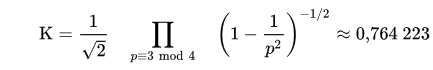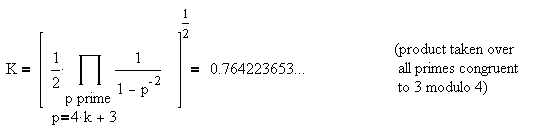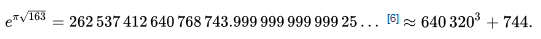## Perl Weekly Challenge # 6: Ramanujan's Constant

These are some answers to Challenge 2 of the Week 6 of the Perl Weekly Challenge organized by Mohammad S. Anwar.

Challenge 1 (compact numeric ranges) was covered in this post.

Challenge 2 was as follows:

Create a script to calculate Ramanujan’s constant with at least 32 digits of precision. Find out more about it here (Wikipedia link).

The Wikipedia link provided in the question concerning this second challenge was apparently changed some time after the challenge was initially posted.

The original link, posted on Monday, April 29, 2019, was pointing to the Landau-Ramanujan Constant, which relates to the sum of two squares theorem.

Then, two days later, on May 1, 2019, I noticed that the link had changed and pointed towards this other Wikipedia page about Ramanujan's constant, which refers to irrational (well, in this case, actually transcendental) numbers that almost look like integers. I do not know when exactly the Wikipedia link was changed.

I guess that my good friend Mohammad Anwar got carried away when writing the challenge because it related to one of his most famous fellow citizens, Indian mathematician Srinivasa Ramanujan (1887-1920). If you've never heard about Ramanujan or don't know much about him, please visit the Wikipedia article just mentioned and search further on the Internet; he is, despite limited access to other mathematicians of the time for a large part of his very short life, one of the greatest mathematicians of the early twentieth century.

I worked on the original link (and the Landau-Ramanujan Constant) for a couple of days, only to find out on May 1, 2019 that the question is now different.

The good thing in this apparent mistake is that I've got two challenges for the price of one. Thank you, dear Mohammad, for the bonus.

## Original Challenge (Landau-Ramanujan Constant)

The Landau-Ramanujan Constant is the only constant that was traditionally associated with the name of Ramanujan, at least until relatively recently.

I'll not explain what this constant is, as the best I would be able to do would be to quote or paraphrase the Wikipedia page on the subject.

The one thing missing in that page though (at least in its English version) is the formula of the Euler product (i.e. an infinite product using prime numbers) to compute the value of this constant. Strangely, the French Wikipedia page on the same subject, which admits being essentially a translation of the English page, has the following formula:It is an infinite product for values of `p` that are prime numbers congruent to 3 mod 4 (i.e. prime numbers in the form 4 * k + 3, with `k` an integer). The first numbers satisfying these properties are: 3, 7, 11, 19, 23, 31, 43, 47, ...

The first digits of the Landau-Ramanujan constant found on this Internet page are:

K = 0.7642236535892206629906987312500923281167905413934...

### A Perl 5 Implementation of the Landau-Ramanujan Constant Calculation

Implementing this formula first requires to build a (long) list of prime numbers and then to keep only those that are congruent to 3 mod 4. Here, I'll simply reuse the `find_primes` subroutine which I wrote for the challenge on 5-smooth numbers in Perl Weekly Challenge # 3. Please refer to that blog post if you want explanations on how it works.

Once we know how to generate a long list of primes, computing the formula above is not too complicated:

``````use strict;
use warnings;
use feature 'say';
use constant largest_num => 100_000;

sub find_primes {
my \$num = 5;
my @primes = (2, 3, 5);
while (1) {
\$num += 2;     # check only odd numbers
last if \$num > largest_num;
my \$limit = int \$num ** 0.5;
my \$num_is_prime = 1;
for my \$prime (@primes) {
last if \$prime > \$limit;
if (\$num % \$prime == 0) {
\$num_is_prime = 0;
last;
}
}
push @primes, \$num if \$num_is_prime;
}
return @primes;
}
my @prime_numbers = find_primes;
my @primes_for_rama = grep { \$_ % 4 == 3 } @prime_numbers;

my \$product = 1;
for my \$p (@primes_for_rama) {
my \$term = (1 - (1 / \$p**2)) **(-1/2);
\$product *= \$term;
}
say \$product / 2**(1/2);
``````

Here, we compute a list (`@prime_numbers`) of all prime numbers below 100,000 and then use a `grep` to keep in `@primes_for_rama` those that are congruent to 3 modulo 4. Finally, we iterate over this array to compute the Euler product.

With primes up to 100,000, we obtain the following result:

``````\$ time perl rama.pl
0.764223499932459

real    0m0.137s
user    0m0.078s
sys     0m0.015s
``````

The first six digits after the decimal point (0.764223) are correct, and the calculation is pretty fast (less than 0.14 sec). If we want more accurate digits, we need a larger list of prime numbers. Let's see how it scales.

``````| ------------ | -------------- | -------------- |
| Primes up to | Correct digits | Run time (sec) |
| ------------ | -------------- | -------------- |
| 100,000      | 0.764223       | 0,137          |
| 1,000,000    | 0.7642236      | 1.109          |
| 10,000,000   | 0.76422365     | 20.237         |
| 100,000,000  | 0.7642236535   | 433.43         |
| ------------ | -------------- | -------------- |
``````

With a ceiling of 100 million for the primes, we're getting 2,880,950 eligible primes and as many terms to multiply, and the run time increases to more that 7 minutes.

We can see in the table above that we have here a perfect example of exponential explosion: each time we multiply the ceiling of our array of prime numbers by 10, we only get roughly one more digit of accuracy on the result. The infinite product is converging extremely slowly, and it gets slower and slower. There is just no way we'll be able to obtain 32 digits, as requested in the original challenge (at least not with this approach).

It is actually worse than that. Since the calculations are performed in floating-point arithmetic, numbers get rounded and, at some point, the value obtained no longer changes. For example, the value of the constant obtained for primes until 100,000,000 is 0.76422365350875 (with the four last digits wrong) and took more than 7 minutes to compute. With primes up to 500,000,000, run time exceeded 74 minutes, and the value obtained (including the four wrong digits at the end) was just exactly the same.

Using the Math::BigRat or Math::BigFloat core modules would certainly make it possible to solve the latter problem, but they would make the first problem even worse, because big numbers arithmetic is generally much slower than normal floating-point arithmetic. There are some ways to alleviate this problem to some extent, but certainly not to the point of getting anywhere near 32 digits in a reasonable amount of time.

I'll leave it at that: I was able to compute relatively easily the first ten digits of the Landau-Ramanujan constant, but computing 32 digits seems to be out of reach. Or, at least, it involves complexities beyond the scope of such a challenge.

### A Perl 6 Implementation of the Landau-Ramanujan Constant Calculation

Perl 6 will make our code much simpler, but we will most probably hit the same limitations as the P5 solution.

For the P6 implementation, I'll use a slightly different version of the Euler product for the Landau-Ramanujan constant, which I found on the Wayback Machine:The reason for choosing this variant of the formula is that it might make it possible to make most of the calculations with simple arithmetic operations on Rats and to postpone the determination of the square root until the last calculation.

``````use v6;

my @primes = grep { .is-prime }, map { 4 * \$_ + 3},  0..1_000_000;
my @terms =  map { 1 / (1 - (1/(\$_ * \$_)) ) }, @primes;
my \$product = ([*] @terms) /2;
say \$product ** (1/2);
``````

The results are essentially the same as with the P5 implementation (0.76422365, i.e. 8 correct digits with an input range of `0..1_000_000` corresponding to 141,643 eligible primes and terms in the product), and 10 correct digits when going for a ten-times larger range.

But it is much slower than the P5 solution. One reason is that a lot of the calculations are done with `RAT` type instead of floats, which means computing things with a much greater accuracy, even if, in that specific case, that greater accuracy doesn't bring any obvious benefit. Another (more important) reason is that, although the `ìs-prime` built-in method is remarkably fast, it still takes a lot of time to execute when you run it a million times. And, I hate to say, Perl 6 is still significantly slower than Perl 5 for such CPU intensive computations.

## Updated Challenge (Ramanujan's Constant)

What has become known as the Ramanujan Constant in the recent period is a number that is an "almost integer" and has in fact little to do with mathematician Srinivasa Ramanujan.

This number is the following one:As you can see, there are nine 9 digits after the decimal point, so that this number, which is built from a formula involving exponentials on one algebraic and two transcendental numbers, almost looks like an integer (when rounded to less than 9 digits after the decimal point).

The number in question had been discovered by mathematician Charles Hermitte in 1859, more than 25 years before Ramanujan’s birth.

The reason why it has become known as Ramanujan’s constant is that, in 1975, "recreational mathematics" columnist Martin Gardner published in Scientific American an April fool article where he claimed that said number, calculated from algebraic and transcendental numbers, was in fact an integer, and further claimed that Ramanujan has already discovered that in the early twentieth century. This was just a joke, as this number is transcendental, but is an impressively close approximation of an integer. At the time, computers were not able to compute this number with enough accuracy to disproof Gardner's assertion. Following that, people have started to call this number Ramanujan’s constant (Ramanujan worked on a number of similar numbers and probably also on this one, but there is no evidence that he discovered anything significantly new on that specific number).

### Perl 5 Solution: Using Big Number Modules

Using regular floating-point arithmetic obviously does not work because it does not provide sufficient accuracy:

``````\$ perl -E '\$pi = 3.14159265358979323846; say exp (\$pi * sqrt 163);'
2.62537412640768e+17
``````

I've been reluctant so far to use external Perl modules for the answers to Perl Weekly Challenge, because I considered that using a module to solve the challenge was sort of cheating a bit. Here, there are two arguments that make me think differently: first, I'm going to use core modules (i.e. modules that are part of the core Perl system), and I am going to use modules that are not giving you a ready-made algorithm, but just provide you with sufficient accuracy.

We can use the Math::BigFloat core module.

``````use strict;
use warnings;
use Math::BigFloat "bpi";
my \$sqrt_163 = Math::BigFloat->new(163)->bsqrt;
my \$big_e =  Math::BigFloat->new(1)->bexp;
printf "%.33s\n", \$big_e ** (bpi() * \$sqrt_163);
``````

which duly prints Ramanujan's constant with an accuracy of 32 significant digits:

``````262537412640768743.99999999999925
``````

Here I wanted to use the usual arithmetic operators (which are overloaded for BigFloat objects), because I thought that it would make the formula more readable, but I have to admit the syntax looks a bit cumbersome. Using chained method invocations makes the code more concise (you should read the code implementing the math formula from right to left):

``````use strict;
use warnings;
use feature "say";
use Math::BigFloat "bpi";
say Math::BigFloat->new(163)->bsqrt->bmul(bpi)->bexp(32);
``````

This outputs Ramanujan's constant just as before:

``````262537412640768743.99999999999925
``````

However, I'm not a great fan of chained method invocations. Using the bigrat core module, which is just a wrapper around other big number libraries, allows a function call syntax and makes the code more concise, so that we can satisfy the requirement with a simple Perl one-liner:

``````\$ perl -Mbigrat=PI,bexp -E 'say bexp(PI * sqrt(163), 32);'
262537412640768743.99999999999925
``````

Note that if we reduce the accuracy to 29 digits (instead of 32):

``````\$ perl -Mbigrat=PI,bexp -E 'say bexp(PI * sqrt(163), 29);'
262537412640768744.00000000000
``````

we obtain a good visual illustration of the reason why Ramanujan's constant is sometimes called an "almost integer".

### Perl 6 Solution Using the Builtin FatRat Type

The Wikipedia page on Ramanujan's constant and the formula given at the beginning of the Update Challenge section of this post show that the integer part of this constant is equal to `640_320 ** 3 + 744` (i.e. 262537412640768744). The Wikipedia article further explains that the difference between this number and Ramanujan's constant is given by:So we just need to apply this formula. Let's do it under the Rakudo REPL:

``````> my \$a = 640_320 ** 3 + 744; # \$a is the integer approximation of R's constant
262537412640768744
> my \$r-constant = \$a - 196844 / \$a;
262537412640768743.999999999999250225
> say \$r-constant.fmt("%.33s");
262537412640768743.99999999999925
``````

Note that we are a bit lucky: the value obtained for `\$r-constant` has an accuracy of 33 digits, and we only need 32. Using the `FatRat` type (instead of the implicit `Rat` type used above) does not improve accuracy, it is the math formula that is an approximation of Ramanujan’s constant.

## Wrapping up

The next week Perl Weekly Challenge is due to start very soon. If you're interested in participating to this challenge, please check https://perlweeklychallenge.org/ and make sure you answer the challenge before 6 p.m. BST (British summer time) on next Sunday, May 12. And, please, also spread the word about the Perl Weekly Challenge if you can.I am the author of the "Think Perl 6" book (O'Reilly, 2017) and I blog about the Perl 5 and Raku programming languages.